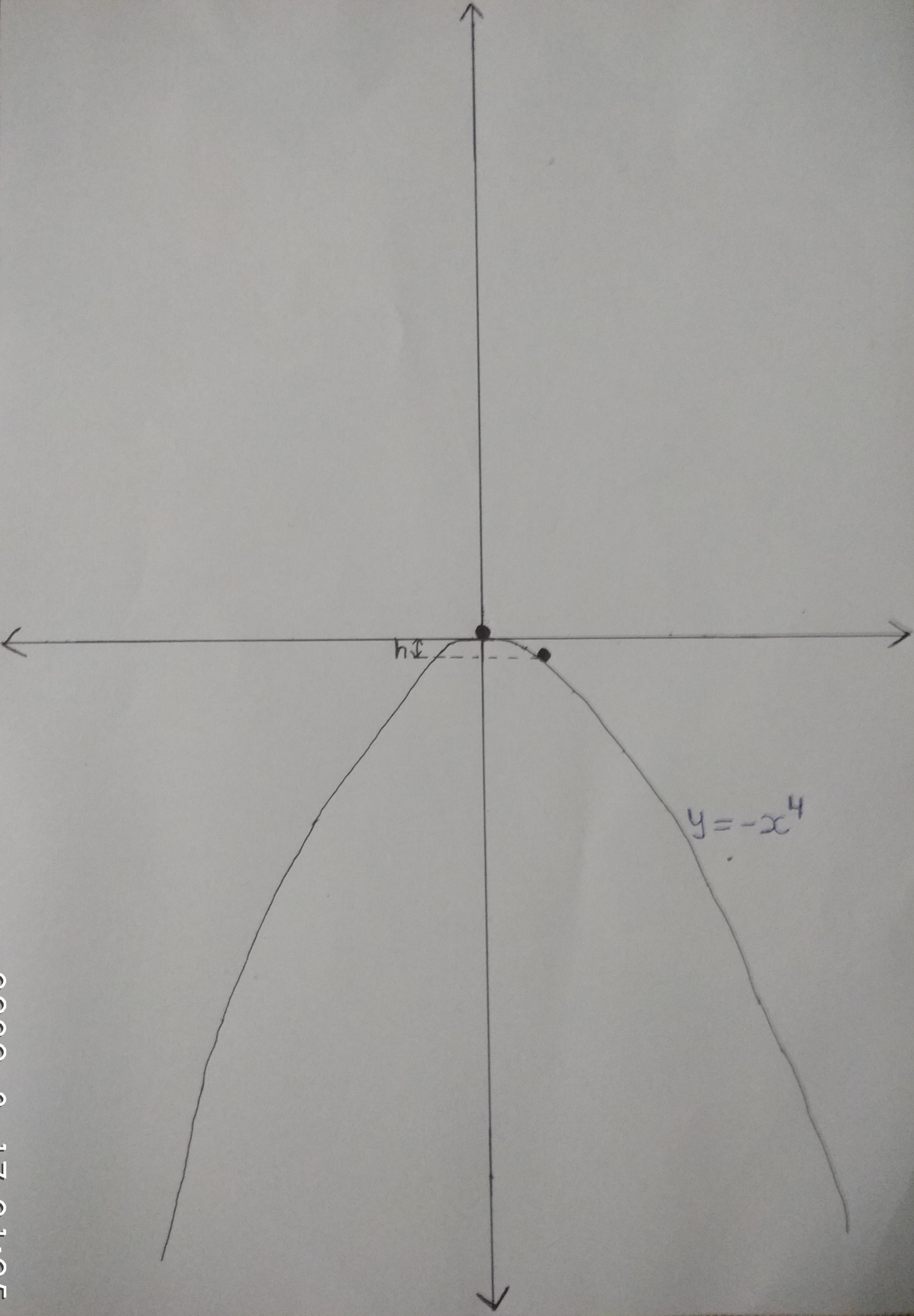# Graphical MechanicsA particle of mass $m$ is released from origin on a wedge shaped as $y = -x^4$ and given a very slight push to the right. After falling through height $h$, it loses contact with the wedge. Find $h$.

If $\displaystyle h = \frac{a}{b} \text{ where } a, b \in \N \text{ and } \gcd(a, b) = 1$, Enter answer as $a + b$.

Details and Assumptions:

• Take acceleration due to gravity $\color{#3D99F6}{g = 10m/s^2}$
• Very slight push means initial velocity $\color{#3D99F6}{u \approx 0}$
• All surfaces are $\color{#3D99F6}{\text{smooth}}$

Inspiration Aniket Sanghi

All of my problems are original

×

Problem Loading...

Note Loading...

Set Loading...